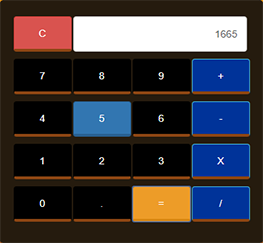## CALCULATOR USING JAVASCRIPT

All our calculator will do is basic arithmetic: add, subtract, multiply and divide. It includes a display area of numbers, numeric buttons 0-9, decimal point button, function buttons(+,-,*,/ & C), and Calculate button ( = ).

Type : Project

File Size : 56.6 KB# Similar Projects and Reports

#### CALCULATOR USING JAVASCRIPT

All our calculator will do is basic arithmetic: add, subtract, multiply and divide. It includes a display area of numbers, numeric buttons 0-9, decimal point button, function buttons(+,-,*,/ & C), and Calculate button ( = ).

#### AUTO SUM CALCULATION USING JAVASCRIPT

This JQuery script will show how to auto calculate sum while you type values in the fields. It’s a Simple Program for beginners. There’s nothing much in this program, user types a certain value in empty TextBox after that, the sum will automatically displ...

#### Calculator In HTML Using JavaScript With Source Code

This Calculator is a simple project in HTML5, CSS, and JavaScript. This project is for solving the mathematical calculations of numbers. You can see the basic features of the calculator in this project.

#### JavaScript Calculator With Source Code

This javascript calculator project is a simple project in HTML5, CSS, and JavaScript. Here, the calculator functions as that of a real-life simple calculator. This project is for solving the mathematical calculations of numbers. You can see the basic feat...

#### Calculator In JavaScript With Source Code

The calculator is a simple project in HTML5, CSS, and JavaScript. Here, the calculator functions as that of a real-life simple calculator. This project is for solving the mathematical calculations of numbers. You can see the basic features of the calculat...

#### Simple JavaScript Calculator With Source Code

This Simple JavaScript calculator project is a simple project in HTML5, CSS, and JavaScript. Here, the calculator functions as that of a real-life simple calculator. This project is for solving the mathematical calculations of numbers. You can see the bas...